# GMAT Math : Rectangles

## Example Questions

← Previous 1 3

### Example Question #61 : Quadrilaterals

Ronald is making a bookshelf with a rectangular base that will be two yards tall. What is the area of the base?

I) The distance around the base will beyards.

II) The smaller sides of the base are half the length of the longer sides.

Both statements together are needed to answer the question.

Statement I is sufficient to answer the question, but Statement II is not sufficient to answer the question.

Statement II is sufficient to answer the question, but Statement I is not sufficient to answer the question.

Either statement alone is sufficient to answer the question.

Both statements together are needed to answer the question.

Explanation:

To find the area we need the length and width of the rectangle. We can use II together with I to make an equation for perimeter with only one unknown.

So we need both to solve.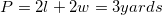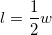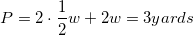Solve for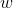and then go back to findand then with that you can find the area of the base and you are finished.

### Example Question #1 : Rectangles

Find a possible width of rectangle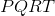.

I)has a perimeter of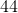fathoms.

II)has a diagonal length offathoms.

Either statement is sufficient to answer the question.

Both statements are needed to answer the question.

Statement II is sufficient to answer the question, but statement I is not sufficient to answer the question.

Statement I is sufficient to answer the question, but statement II is not sufficient to answer the question.

Both statements are needed to answer the question.

Explanation:

When asked to find the width of a rectangle we will need to use both statemests together.

For Statement I) we can use the perimeter formula.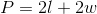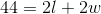Now, for Statement II) we will use the length of the diagonal along with the Pythagorean Theorem.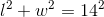From here you can solve the perimeter equation in terms of either l or w. Then you can use substitution into the Pythagorean Theorem to solve for a possible width.

### Example Question #1 : Rectangles

Find the length of the side of a rectangle with a width three times the length.

1. The area of the rectangle is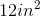.
2. The perimeter of the rectangle is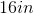Statement 1 alone is sufficient, but statement 2 alone is not sufficient to answer the question.

Both statements taken together are sufficient to answer the question, but neither statement alone is sufficient.

Statement 2 alone is sufficient, but statement 1 alone is not sufficient to answer the question.

Statements 1 and 2 are not sufficient, and additional data is needed to answer the question.

Each statement alone is sufficient to answer the question.

Each statement alone is sufficient to answer the question.

Explanation:

Statement 1: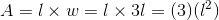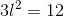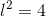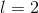Statement 1 is sufficient to answer the question

Statement 2: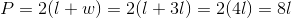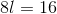Statement 2 is also sufficient to answer the question

### Example Question #1 : Dsq: Calculating The Length Of The Side Of A Rectangle

A rectangle has a width measuring twice the length. Find the length.

1. The rectangle has a perimeter of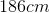.
2. The rectangle's area is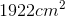.

Both statements taken together are sufficient to answer the question, but neither statement alone is sufficient.

Each statement alone is sufficient to answer the question.

Statements 1 and 2 are not sufficient, and additional data is needed to answer the question.

Statement 1 alone is sufficient, but statement 2 alone is not sufficient to answer the question.

Statement 2 alone is sufficient, but statement 1 alone is not sufficient to answer the question.

Each statement alone is sufficient to answer the question.

Explanation:

Statement 1:

Recall the formula to find the perimeter of a rectangle. Substitute in the given information and solve.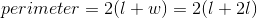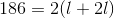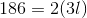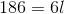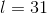Statement 2:

Recall the formula for the area of a rectangle. Substitute in the given information and solve.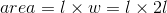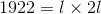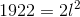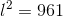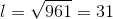Each statement alone is sufficient to answer the question.

### Example Question #5 : Rectangles

Given parallelogramwith diagonal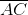. Is this parallelogram a rectangle?

1)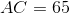2)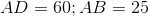BOTH statements TOGETHER are insufficient to answer the question.

BOTH statements TOGETHER are sufficient to answer the question, but NEITHER statement ALONE is sufficient to answer the question.

Statement 2 ALONE is sufficient to answer the question, but Statement 1 ALONE is NOT sufficient to answer the question.

EITHER statement ALONE is sufficient to answer the question.

Statement 1 ALONE is sufficient to answer the question, but Statement 2 ALONE is NOT sufficient to answer the question.

BOTH statements TOGETHER are sufficient to answer the question, but NEITHER statement ALONE is sufficient to answer the question.

Explanation:

The length of one diagonal alone does not prove the parallelogram to be a rectangle, nor do the lengths of the sides.

Suppose we know all of these lengths, though. Sinceis a parallelogram, if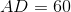, then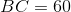The sides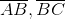and diagonalform a triangle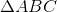with sidelengths 25, 60, and 65. The parallelogram is a rectangle if and only if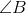is a right angle; therefore, we must determine whether the conditions of the Pythagorean Theorem hold: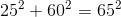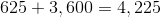This is true;is a right angle andis a rectangle.

Therefore, both statements together are sufficient to answer the question, but neither statement alone is sufficient to answer the question.

### Example Question #64 : Quadrilaterals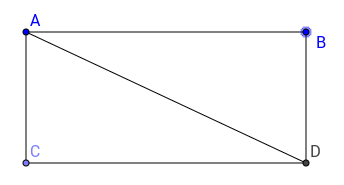What is the length of the diagonal of rectangle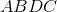(1)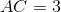(2)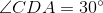and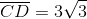Statement 1 alone is sufficient

Statements 1 and 2 together are not sufficient

Both statements together are sufficient

Each statement alone is sufficient

Statement 2 alone is sufficient

Statement 2 alone is sufficient

Explanation:

In order to find the diagonal, we must know the sides of the rectangle or know whether the triangles ADC or ABD have special angles.

Statement 1 alone doesn't let us calculate the hypothenuse of the triangles, because we only know one side.

Statement 2 alone is sufficient because it allows us to find all angles of the triangles inside of the rectangle. We can see that they are special triangles with angles 30-60-90. Any triangle with these angles will have its sides in ratio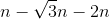, where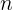is a constant. Here,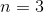, knowing this, we can calculate the length of the hypothenuse, also the diagonal, which will be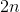.

Hence, statement 2 is sufficient.

### Example Question #101 : Geometry

Rectangle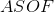has a perimeter of, what is its area?

I) The diagonal ofis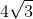inches.

II) The length of one side isinches.

Either statement alone is sufficient to answer the question.

Both statements together are needed to answer the question.

Statement I is sufficient to answer the question, but Statement II is not sufficient to answer the question.

Statement II is sufficient to answer the question, but Statement I is not sufficient to answer the question.

Statement II is sufficient to answer the question, but Statement I is not sufficient to answer the question.

Explanation:

I) Gives us the length of ASOF's diagonal. This by itself does not give us any way of finding the other sides.

II) Gives us one side length. From there we can use the perimeter to find the other side length and then the area.

Therefore, Statement II is sufficient to answer the question.

### Example Question #1 : Dsq: Calculating The Length Of The Diagonal Of A RectangleRectangle, has diagonal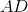. What is the length of?

(1) Angle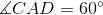.

(2)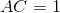.

Statement 2 alone is sufficient.

Statement 1 alone is sufficient.

Statements 1 and 2 together are not sufficient.

Both statements together are sufficient.

Each statement alone is sufficient.

Both statements together are sufficient.

Explanation:

The length of a diagonal of a rectangle can be calculated like a hypotenuse using the Pythagorean Theorem provided we have information about the lengths of the rectangle.

Statement 1 tells us that both triangles ADC and ABD have their angles in ratio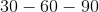, which means that their sides will have length in ratio, whereis a constant. We can't tell however what length the diagonal will be.

Statment 2 tells us that side AC is 1. From there we can't conclude anything. Indeed, rectangle ABCD might as well be a square or a very thin rectangle, we don't know.

Both statements together however, allow us to tell that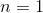and therefore that the diagonal will be 2.

Hence, both statements together are sufficient.

### Example Question #4 : Dsq: Calculating The Length Of The Diagonal Of A Rectangleis a rectangle. What is the ratio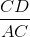?

(1)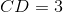.

(2)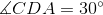.

Both statements together are sufficient.

Statement 2 alone is sufficient.

Statement 1 and 2 together are not sufficient.

Each statement alone is sufficient.

Statement 1 alone is sufficient.

Statement 2 alone is sufficient.

Explanation:

To solve this, we need information about the lengths of the sides or to whether triangles ADB and ACD are special triangles.

Statement 1 tells us that CDA has length 3. This is not enough and we still don't know whether the rectangle is of a special type of rectangle.

Statement 2 tells us that triangles ADB and ACD are special triangles, indeed, they have their angles in ratio. That means that their sides will be in ratio. Now we don't need to know what is constant, since it will cancel out in the ratio.

Therefore, statement 2 alone is sufficient.

### Example Question #1 : Dsq: Calculating The Length Of The Diagonal Of A Rectangle

Find the diagonal of rectangle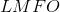.

I) The area ofis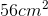.

II) The perimeter ofis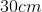.

Both statements are needed to answer the question.

Either statement is sufficient to answer the question.

Statement II is sufficient to answer the question, but statement I is not sufficient to answer the question.

Statement I is sufficient to answer the question, but statement II is not sufficient to answer the question.

Both statements are needed to answer the question.

Explanation:

In order to find the diagonal of a rectangle we need the length of both sides.

We can't find the lengths with just the area or just the perimeter, but by using them both together we can make a small system of equations with two unknowns and two equations.

Then we can solve for each side and use the Pythagorean Theorem to find our diagonal.

← Previous 1 3

### All GMAT Math Resources